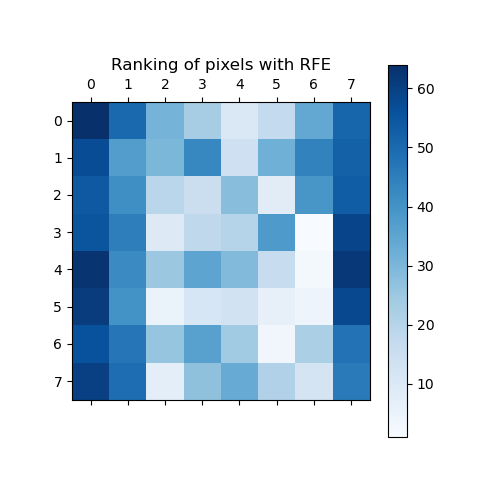# Recursive feature elimination¶

A recursive feature elimination example showing the relevance of pixels in a digit classification task.

Notefrom sklearn.svm import SVC
from sklearn.feature_selection import RFE
import matplotlib.pyplot as plt

X = digits.images.reshape((len(digits.images), -1))
y = digits.target

# Create the RFE object and rank each pixel
svc = SVC(kernel="linear", C=1)
rfe = RFE(estimator=svc, n_features_to_select=1, step=1)
rfe.fit(X, y)
ranking = rfe.ranking_.reshape(digits.images.shape)

# Plot pixel ranking
plt.matshow(ranking, cmap=plt.cm.Blues)
plt.colorbar()
plt.title("Ranking of pixels with RFE")
plt.show()


Total running time of the script: ( 0 minutes 3.911 seconds)

Gallery generated by Sphinx-Gallery